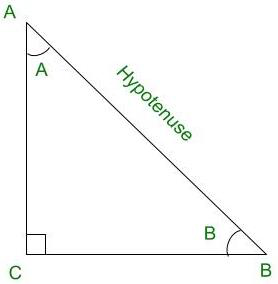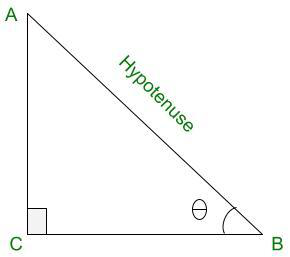Open in App
Not now

# If x = a sin θ + b cos θ and y = a cos θ – b sin θ, then prove that x2 + y2 = a2 + b2.

• Last Updated : 21 Sep, 2021

Trigonometry is the relation between the angles and sides of a right-angled triangle. In a right angles triangle, there are 3 angles of which one angle is a right angle (90°) and other two angles are acute angles and there are 3 sides. The side opposite to the right angle is called Hypotenuse. There are 6 ratios between these sides based on the angle between them and they are called Trigonometric Ratios.

The 6 Trigonometric ratios are:

• Sine (sin)
• Cosine (cos)
• Tangent (tan)
• Cosecant (cosec)
• Secant (sec)
• Cotangent (cot)Right-angled triangle ACB

Sine (sin):

Sine of an angle is defined by the ratio of lengths of sides which is opposite to the angle and the hypotenuse. For the above triangle, the value of angle sine is given for both ∠A and ∠B, the definition for sine angle is the ratio of the perpendicular to its hypotenuse.Cosine (cos):

Cosine of an angle is defined by the ratio of lengths of sides which is adjacent to the angle and the hypotenuse. For the above triangle, the value of the angle cos is given for both ∠A and ∠B, the definition for cos angle is the ratio of the base to its hypotenuse.Tangent (tan):

The tangent of an angle is defined by the ratio of the length of sides which is opposite to the angle and the side which is adjacent to the angle. For the above triangle, the value of the angle tan is given for both ∠A and ∠B, the definition for tan angle is the ratio of the perpendicular to its base.Cosecant (cosec):

The cosecant of an angle is defined by the ratio of the length of the hypotenuse and the side opposite the angle. For the above triangle, the value of the angle cosec is given for both ∠A and ∠B, the definition for cosec angle is the ratio of the hypotenuse to its perpendicular.Secant (sec):

Secant of an angle is defined by the ratio of the length of the hypotenuse and the side and the side adjacent to the angle. For the above triangle, the value of the angle sec is given for both ∠A and ∠B, the definition for sec angle is the ratio of the hypotenuse to its base.Cotangent (cot):

The cotangent of an angle is defined by the ratio of the length of sides that is adjacent to the angle and the side which is opposite to the angle. For the above triangle, the value of the angle cot is given for both ∠A and ∠B, the definition for cot angle is the ratio of the hypotenuse to its base.### If x = a sin θ + b cos θ and y = a cos θ –b sin θ, then prove thatx2 + y2 = a2 + b2.

Solution:Right-angled triangle ACB

x = asinθ + bcosθ

Squaring both sides we get,

x2 = (asinθ + bcosθ)2x2 = a2sin2θ + 2absinθcosθ + b2cos2θ ………. ( 1 )

y = acosθ – bsinθ

Squaring both sides we get,

y2 = (acosθ – bsinθ)2y2 = a2cos2θ – 2absinθcosθ + b2sin2θ ………. ( 2 )

Adding (1) and (2), we get,

x2 + y2 = (a2sin2θ + 2absinθcosθ + b2cos2θ) + (a2cos2θ – 2absinθcosθ + b2sin2θ)x2 + y2 = a2(sin2θ + cos2θ) + b2(sin2θ + cos2θ)

We know that,sin2θ + cos2θ = 1x2 + y2 = a2 + b2

Hence, Proved.

### Similar Problems

Question 1: If x = acosθ and y = bsinθ, Thenb2x2 + a2y2 = a2b2

Solution:

x = acosθ, y = bsinθ ……….. ( 1 )

L.H.S =b2x2 + a2y2L.H.S = b2(acosθ)2 + a2(bsinθ)2 …….(From(1))L.H.S = a2b2cos2θ + a2b2sin2θL.H.S = a2b2(cos2θ + sin2θ)

We know that,sin2θ + cos2θ = 1L.H.S = a2b2L.H.S = R.H.Sb2x2 + a2y2 = a2b2

Hence, Proved.

Question 2: If x = asinθ and y = btanθ, then prove thatSolution:

x = asinθ, y = btanθ ……….. ( 1 )………. ( From (1) )Since, sin2θ + cos2θ = 1 ⇒ sin2θ = 1 – cos2θL.H.S = 1L.H.S = R.H.SHence, Proved.

Question 3: Prove the following: If x=asecθ + btanθ and y=atanθ + bsecθ, prove thatx2 − y2 = a2 − b2

Solution:

x=asecθ + btanθ and y = atanθ + bsecθ ……….. ( 1 )

L.H.S = x2 − y2

From (1), we get

L.H.S = (asecθ + btanθ)2 – (atanθ + bsecθ)2L.H.S = (a2sec2θ + 2absecθtanθ + b2tan2θ) – (a2tan2θ + 2absecθtanθ + b2sec2θ)L.H.S = a2sec2θ + 2absecθtanθ + b2tanθ2 – a2tan2θ – 2absecθtanθ – b2sec2θL.H.S = a2(sec2θ – tan2θ) – b2(sec2θ – tan2θ)

We know that, sec2θ – tan2θ =1L.H.S = a2(1) – b2(1)L.H.S = a2 – b2L.H.S = R.H.Sx2 − y2 = a2 − b2

Hence, Proved.

My Personal Notes arrow_drop_up